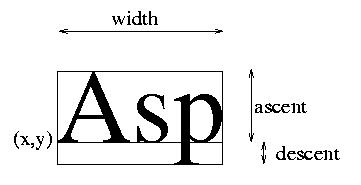## Using font metrics: framechars.c

Drawing strings in random positions of the screen is easy, as we do not have to care much about how large and high the string is. In many cases, however, this information is important. We consider an example similar to the one of the previous page, still writing strings in random positions of the screen, but framing them into boxes. The difficulty is only that we have to know how large and high the string is.

In order to determine this information, we need two additional variables. The first one is a font structure variabile, of type XFontStruct, and contains information about a font. The second one is only needed to determine the first one.

```  XFontStruct *fs;
XGCValues v;
```
Right after the font is loaded, we can find the font structure:
```  /* get font metrics */
XGetGCValues (dpy, g, GCFont, &v);
fs = XQueryFont (dpy, v.font);
```
To check how large a string will be on the screen, we use the function XTextWidth, which takes as arguments the font structure, a string, and its lenght. Note that many fonts are non-proportional, that is, the characters do not all have the same width. This is why it does not make sense-in general-to ask for the widht of a font.

The height of the font is given as two numbers: the first one tells how high the font will raise over its feet, the second one tells how low it may goes under the feet. These two numbers are the fields ascent and descent of the font strucuture.The completed module does not only draw a string in a random position. It first stores the random position into two variabiles x and y, then determines the size of the string, and draws the string and the frame around it. We declare x, y, and txtwidth to be integer variabiles, and then modify the string drawing fragment as follows. The module framechars.c draws two boxes around the strings: the first one is the ``upper'' part of the characters (above the feet), while the second one is below.

```      /* random x and y position */
x=random()%wa.width;
y=random()%wa.height;

/* get text width and height */
txtwidth=XTextWidth (fs, "Ooops!", strlen("Ooops!") );

/* draw the frames (boxes) */
XDrawRectangle(dpy, root, g, x, y-fs->ascent, txtwidth, fs->ascent);
XDrawRectangle(dpy, root, g, x, y, txtwidth, fs->descent);

/* draw a string */
XDrawString(dpy, root, g, x, y,
"Ooops!", strlen("Ooops!") );
```

A more intersting example is metrics.c, that fills the whole screen with the same string, writing it in lines.

Next: using images.